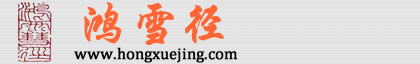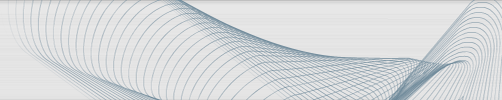# IE浏览器javascript new date NAN bug的解决

```function addDate(date,days){
var d=new Date(date);
d.setDate(d.getDate()+days);
var month=d.getMonth()+1;
var day = d.getDate();
if(month<10){
month = "0"+month;
}
if(day<10){
day = "0"+day;
}
var val = d.getFullYear()+"-"+month+"-"+day;
return val;
}```

```function addDate(date,days){
var d=new Date(date.replace("-","/"));
d.setDate(d.getDate()+days);
var month=d.getMonth()+1;
var day = d.getDate();
if(month<10){
month = "0"+month;
}
if(day<10){
day = "0"+day;
}
var val = d.getFullYear()+"-"+month+"-"+day;
return val;
}```

暂无相关信息